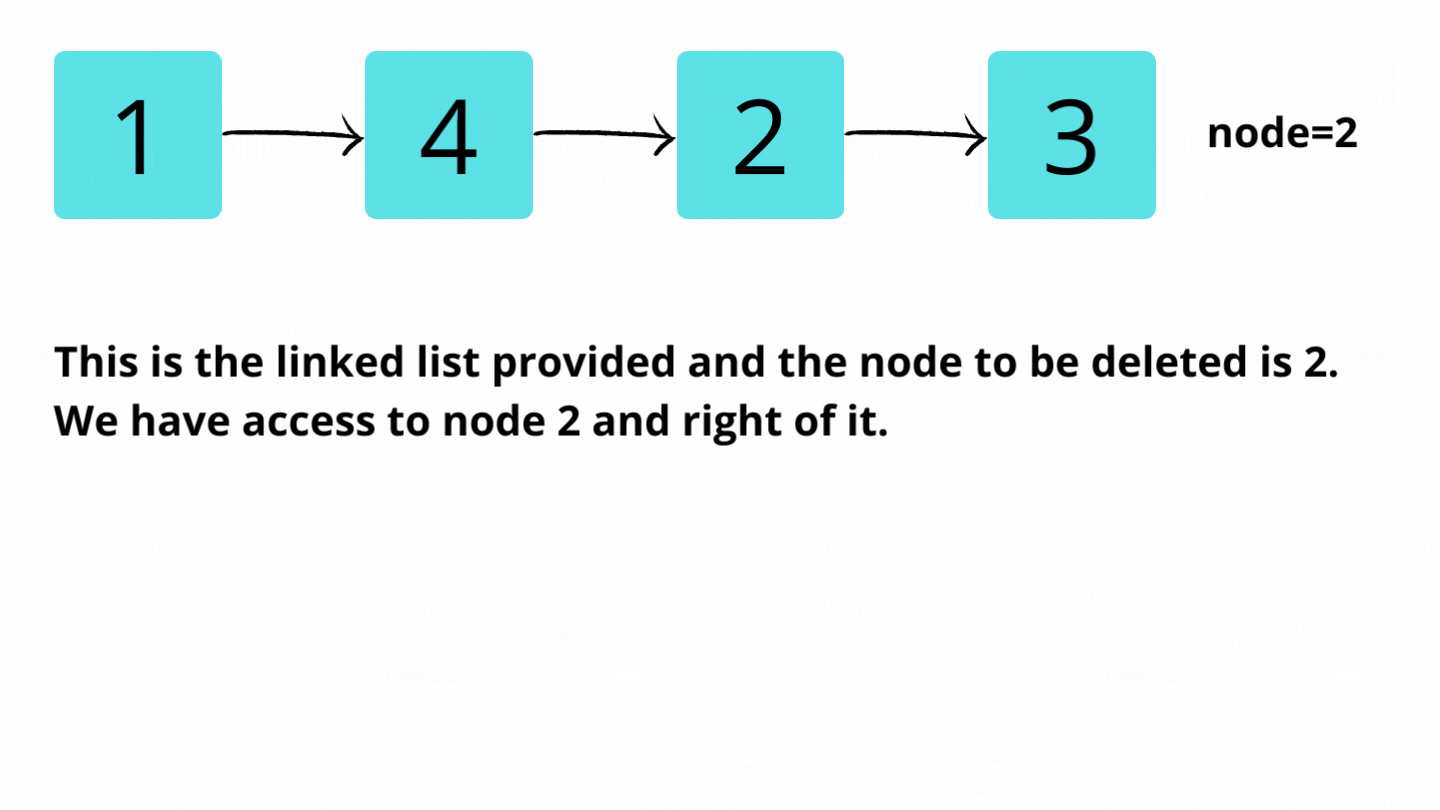# Delete given node in a Linked List : O(1) approach

Problem Statement: Write a function to delete a node in a singly-linked list. You will not be given access to the head of the list instead, you will be given access to the node to be deleted directly. It is guaranteed that the node to be deleted is not a tail node in the list.

Examples:

```Example 1:
Input:
Node = 2
Output:
Explanation: Access is given to node 2. After deleting nodes, the linked list will be modified to [1,4,3].

Example 2:
Input:
Node = 1
Output: Linked list: [2,3,4]
Explanation:
Access is given to node 1. After deleting nodes, the linked list will be modified to [2,3,4].```

Solution:

DisclaimerDon’t jump directly to the solution, try it out yourself first.

Approach:

We are given access to nodes that we have to delete from the linked list. So, whatever operation we want to do in the linked list, we can operate in the right part of the linked list from the node to be deleted.

The approach is to copy the next node’s value in the deleted node. Then, link node to next of next node. This does not delete that node but indirectly it removes that node from the linked list.

Dry Run:Code:

## C++ Code

``````#include<iostream>
using namespace std;

class node {
public:
int num;
node* next;
node(int a) {
num = a;
next = NULL;
}
};
//function to insert node at the end of the list
void insertNode(node* &head,int val) {
node* newNode = new node(val);
if(head == NULL) {
return;
}
node* temp = head;
while(temp->next != NULL) temp = temp->next;
temp->next = newNode;
}
//function to get reference of the node to delete
node* getNode(node* head,int val) {

}
//delete function as per the question
void deleteNode(node* t) {
t->num = t->next->num;
t->next = t->next->next;
return;
}
//printing the list function
void printList(node* head) {
while(head->next != NULL) {
}
}

int main() {
node* head = NULL;
//inserting node
//printing given list
node* t = getNode(head,2);
//delete node
deleteNode(t);
//list after deletion operation
cout<<"Linked List after deletion:\n";
return 0;
}
``````

Output:

1->4->2->3
Linked List after deletion:
1->4->3

Time Complexity: O(1)

Reason: It is a two-step process that can be obtained in constant time.

Space Complexity: O(1)

Reason: No extra data structure is used.

## Java Code

``````import java.util.*;
class Node {
int num;
Node next;
Node(int a) {
num = a;
next = null;
}
}
class TUF{
//function to insert node at the end of the list
static Node insertNode(Node head,int val) {
Node newNode = new Node(val);
if(head == null) {
}
Node temp = head;
while(temp.next != null) temp = temp.next;
temp.next = newNode;
}
//function to get reference of the node to delete
static Node getNode(Node head,int val) {
return null;

}
//delete function as per the question
static void deleteNode(Node t) {
if(t==null)
return;
t.num = t.next.num;
t.next = t.next.next;
return;
}
//printing the list function
static void printList(Node head) {
return;
}
}

public static void main(String args[]) {
Node head = null;
//inserting node
//printing given list
System.out.println("Given Linked List: ");
Node t = getNode(head,2);
//delete node
deleteNode(t);
//list after deletion operation
System.out.println("Linked List after deletion: ");
}
}
``````

Output: